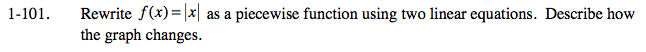### Home > CALC > Chapter 1 > Lesson 1.3.1 > Problem1-101

1-101.

Rewrite f(x) = |x| as a piecewise function using two linear equations. Describe how the graph changes. 1-101 HW eTool (Desmos). Homework Help ✎Start by sketching a graph. If you do not know what y = |x| looks like, graph y = x, then...

How would the absolute value sign change this function?

Notice that x = 0 is the boundary point of y = |x|. So the piecewise function will have two pieces that meet at x = 0. The first piece looks like y = _______ and the second piece looks like y = ___________.

Use the eTool below to view the graphs of the function.
Click the link at right for the full version of the eTool: Calc 1-101 HW eTool